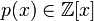# Bunyakovsky conjecture

## Statement

The conjecture (not yet proved or disproved) states the following: supposeis irreducible, i.e.,is an irreducible polynomial of degree two or higher with integer coefficients. Consider the set:Then, one of these two cases must hold:

1. The greatest common divisor of all the elements ofis greater than 1, i.e., all elements ofhave a nontrivial common factor.
2.contains infinitely many prime numbers.

Note that the first case occurs if and only if the polynomial can be written as (a positive integer greater than 1) times (an integer-valued polynomial).

## Related facts and conjectures

### Related conjectures

• Bateman-Horn conjecture is a stronger conjecture that also makes assertions about the frequency of primes in the set of values taken by a polynomial.